# Problem 58997. Compute the water table elevation in an unconfined aquifer with recharge

Problem statement
Write a function to compute the water table elevation h above the bottom of the aquifer and the position of a groundwater divide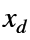given the water table elevations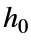at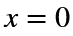,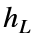at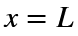, hydraulic conductivity K, and recharge rate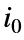. If there is no groundwater divide, set xd to the empty set [].
Background
As in other problems in this series, the movement of groundwater is governed by conservation of mass and (if the flow is slow enough) Darcy’s law. In an unconfined aquifer, the latter relates the flow of water to the gradient in water table elevation above the bottom of the aquifer bywhere w is the width of the aquifer. For steady, one-dimensional flow, conservation of mass says that the flow at any position x is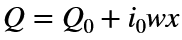where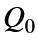is the (unknown) flow at. Using Darcy’s law to replace Q provides a differential equation that can be integrated using the water table elevations at the boundaries to determineand a constant of integration.
Unlike the flows in Cody Problem 58966, the flows here can have a groundwater divide, or a point in the profile that separates flow to the left from flow to the right.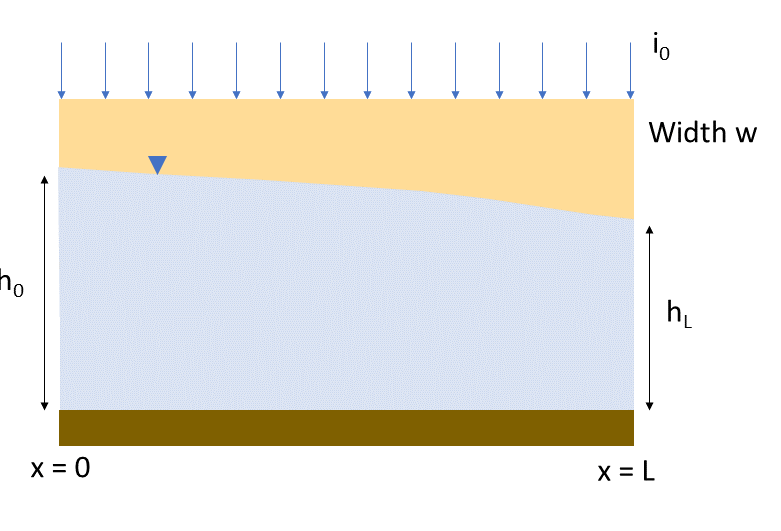### Solution Stats

66.67% Correct | 33.33% Incorrect
Last Solution submitted on Sep 28, 2023

### Community Treasure Hunt

Find the treasures in MATLAB Central and discover how the community can help you!

Start Hunting!